Python‎ > ‎

### A Complete Guide to Time Series Data Visualization in Python

posted Nov 24, 2020, 7:35 AM by Chris G   [ updated Nov 24, 2020, 7:41 AM ]
From: https://towardsdatascience.com/a-complete-guide-to-time-series-data-visualization-in-python-da0ddd2cfb01

## This Should Give You Enough Resources to Make Great Visuals with Time Series Data

Time series data is very important in so many different industries. It is especially important in research, financial industries, pharmaceuticals, social media, web services, and many more. Analysis of time series data is also becoming more and more essential. What is better than some good visualizations in the analysis. Any type of data analysis is not complete without some visuals. Because one good plot can provide you with a better understanding than a 20-page report. So, this article is all about time-series data visualization.

I will start with some very simple visualization and slowly will move to some advanced visualization techniques and tools

I need to make one more thing clear before starting.

‘The complete guide” in the title does not mean, it has all the visualization. There are so many visualizations available in so many different libraries that it is even not practical to have all of them in one article.

But this article should provide you with enough tools and techniques to tell a story or understand and visualize a time series data clearly. I tried to explain some simple and easy ones and some advanced techniques.

## rashida048/Datasets

### Permalink GitHub is home to over 50 million developers working together to host and review code, manage projects, and…

#### github.com

This is a stock dataset. Let’s import some necessary packages and the dataset:

`import pandas as pdimport numpy as npimport matplotlib.pyplot as pltdf = pd.read_csv("stock_data.csv", parse_dates=True, index_col = "Date")df.head()`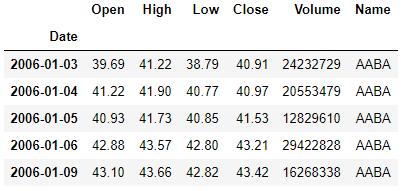I used the ‘parse_dates’ parameter in the read_csv function to convert the ‘Date’ column to the DatetimeIndex format. Most of the time, Dates are stored in string format which is not the right format for time series data analysis. When it is in the DatetimeIndex format, it is a lot helpful to deal with as a time series data. You will see it soon.

I have a detailed article on Time-series data analysis. If you are new to time series data, it will be helpful if you have a look at this article first:

## An Ultimate Guide to Time Series Analysis in Pandas

### All the Pandas Function You Need to Perform Time Series Analysis in Pandas. You Can Use This as a Cheat Sheet as Well.

#### towardsdatascience.com

I explained some important Pandas function in the article above that will be used in this article. Though I will provide a brief idea here as well. But if you need an example to understand better, please feel free to have a look at that previous article.

## Basic Plots First

As I said before, I want to start with some basic plots. The most basic plot should be a line plot using Pandas. I will plot the ‘Volume’ data here. See how it looks:

`df['Volume'].plot()`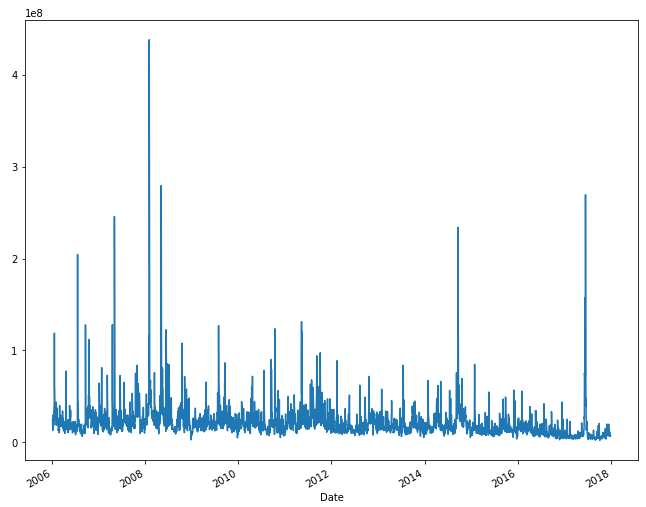This is our plot of ‘Volume’ data that looks pretty busy with some big spikes. It will be a good idea to plot all the other columns as well in a plot to examine the curves of all of them at the same time.

`df.plot(subplots=True, figsize=(10,12))`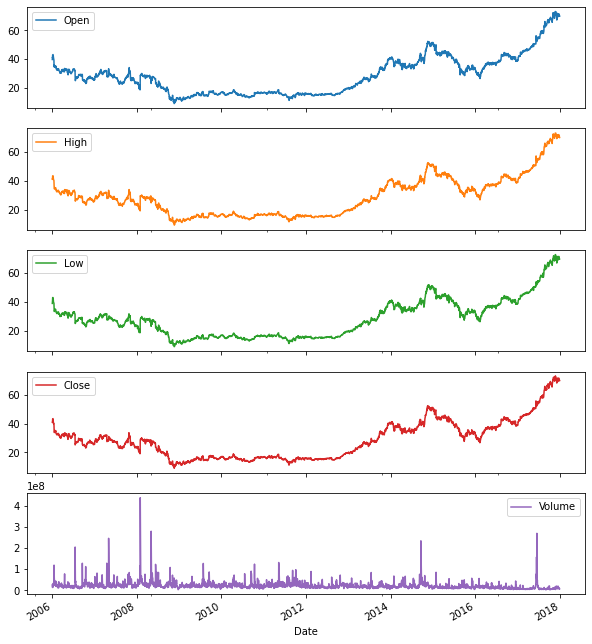The shape of the curve for ‘Open’, ‘Close’, ‘High’ and ‘Low’ data have the same shape. Only the ‘Volume’ has a different shape.

Seasonality

The line plot I used above is great for showing seasonality. Resampling for months or weeks and making bar plots is another very simple and widely used method of finding seasonality. Here I am making a bar plot of month data for 2016 and 2017. For the index, I will use [2016:]. Because our dataset contains data until 2017. So, 2016 to end should bring 2016 and 2017.

`df_month = df.resample("M").mean()fig, ax = plt.subplots(figsize=(10, 6))ax.xaxis.set_major_formatter(mdates.DateFormatter('%Y-%m'))ax.bar(df_month['2016':].index, df_month.loc['2016':, "Volume"], width=25, align='center'`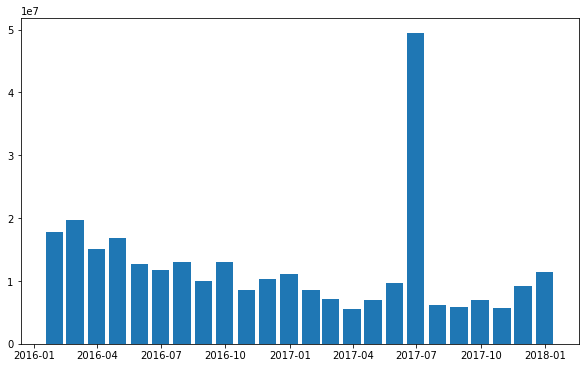There are 24 bars. Each bar represents a month. A huge spike in July 2017. Otherwise, there is no monthly seasonality here.

One way to find seasonality is by using a set of boxplots. Here I am going to make boxplots for each month. I will use ‘Open’, ‘Close’, ‘High’ and ‘Low’ data to make this plot.

`import seaborn as sns#start, end = '2016-01', '2016-12'fig, axes = plt.subplots(4, 1, figsize=(10, 16), sharex=True)for name, ax in zip(['Open', 'Close', 'High', 'Low'], axes):    sns.boxplot(data = df, x='Month', y=name, ax=ax)    ax.set_ylabel("")    ax.set_title(name)    if ax != axes[-1]:        ax.set_xlabel('')`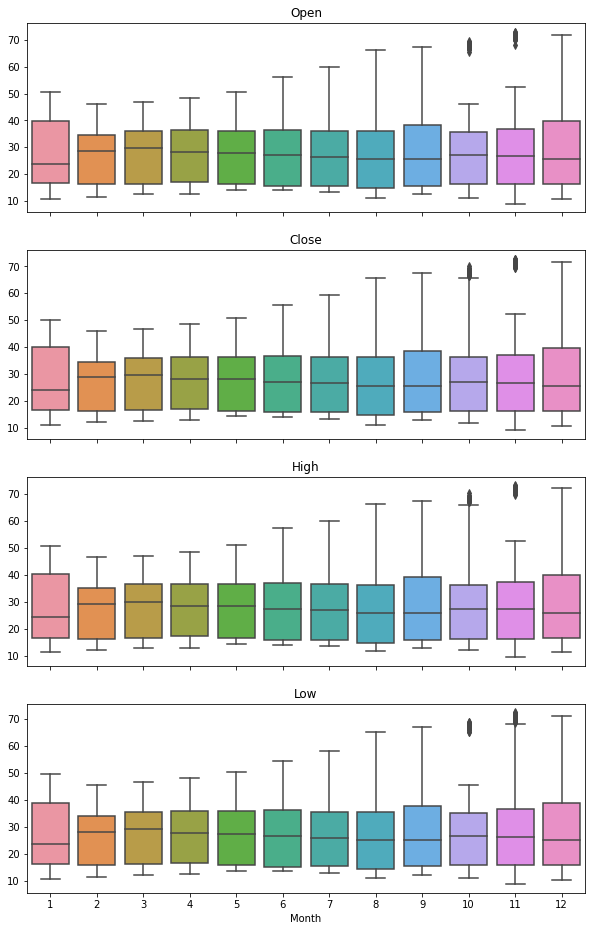It shows the monthly difference in values clearly.

There are more ways to show seasonality. I discussed it one more way at the end.

## Resampling and Rolling

Remember that first line plot of ‘Volume’ data above. As we discussed before, it was too busy. It can be fixed by resampling. Instead of plotting daily data, plotting monthly average will fix this issue to a large extent. I will use the df_month dataset I prepared already for the bar plot and box plots above for this.

`df_month['Volume'].plot(figsize=(8, 6))`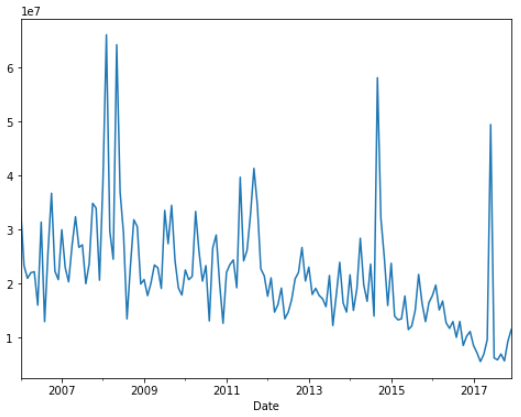Much more understandable and clearer! It gives a better idea about a trend in long term.

Resampling is very common in time-series data. Most of the time resampling is done to a lower frequency.

So, this article will only deal with the resampling of lower frequencies. Though resampling of higher frequency is also necessary especially for modeling purposes. Not so much in data analysis purpose.

In the ‘Volume’ data we are working on right now, we can observe some big spikes here and there. These types of spikes are not helpful for data analysis or for modeling. normally to smooth out the spikes, resampling to a lower frequency and rolling is very helpful.

Now, plot the daily data and weekly average ‘Volume’ in the same plot. First, make a weekly average dataset using the resampling method.

`df_week = df.resample("W").mean()`

This ‘df_week’ and ‘df_month’ will be useful for us in later visualization as well.

Let’s plot the daily and weekly data in the same plot.

`start, end = '2015-01', '2015-08'fig, ax = plt.subplots()ax.plot(df.loc[start:end, 'Volume'], marker='.', linestyle='-', linewidth = 0.5, label='Daily', color='black')ax.plot(df_week.loc[start:end, 'Volume'], marker='o', markersize=8, linestyle='-', label='Weekly', color='coral')label='Monthly', color='violet')ax.set_ylabel("Open")ax.legend()`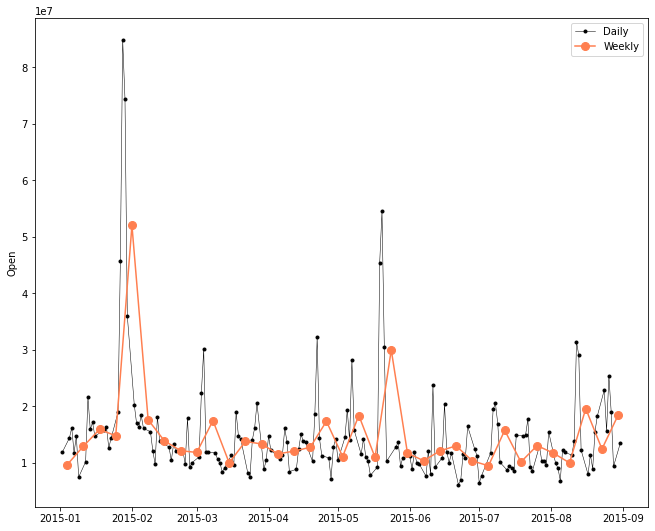Look, the weekly average plot has smaller spikes than daily data.

Rolling is another very helpful way of smoothing out the curve. It takes the average of a specified amount of data. If I want a 7-day rolling, it gives us the 7-d average data.

Let’s include the 7-d rolling data in the above plot.

`df_7d_rolling = df.rolling(7, center=True).mean()start, end = '2016-06', '2017-05'fig, ax = plt.subplots()ax.plot(df.loc[start:end, 'Volume'], marker='.', linestyle='-',         linewidth=0.5, label='Daily')ax.plot(df_week.loc[start:end, 'Volume'], marker='o', markersize=5,         linestyle='-', label = 'Weekly mean volume')ax.plot(df_7d_rolling.loc[start:end, 'Volume'], marker='.', linestyle='-', label='7d Rolling Average')ax.set_ylabel('Stock Volume')ax.legend()`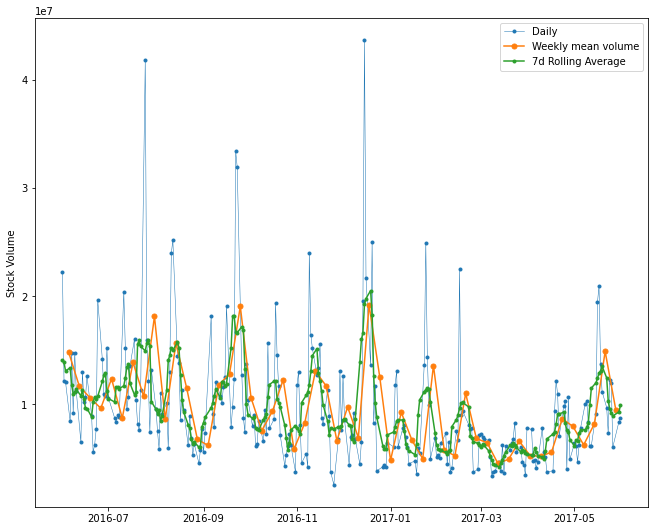A lot going on in this one plot. But If you look at it carefully it is still understandable. If you notice 7-d rolling average is a bit smoother than the weekly average.

It is also common to take a 30-d or 365-d rolling average to make the curve smoother. Please try it yourself.

## Plotting the Change

Lots of time it is more useful to see how the data change over time instead of just everyday data.

There are a few different ways to calculate and visualize the change in data.

Shift

The shift function shifts the data before or after the specified amount of time. If I do not specify the time it will shift the data by one day by default. That means you will get the previous day's data. In financial data like this one, it is helpful to see previous day data and today's data side by side.

`df['Change'] = df.Close.div(df.Close.shift())df['Change'].plot(figsize=(20, 8), fontsize = 16)`

In the code above, .div() helps to fill up the missing data. Actually, div() means division. df. div(6) will divide each element in df by 6. But here I used ‘df.Close.shift()’. So, Each element of df will be divided by each element of ‘df.Close.shift()’. We do this to avoid the null values that are created by the ‘shift()’ operation.

If it is not yet clear to you, please look at the article I mentioned in the beginning.

Here is the output: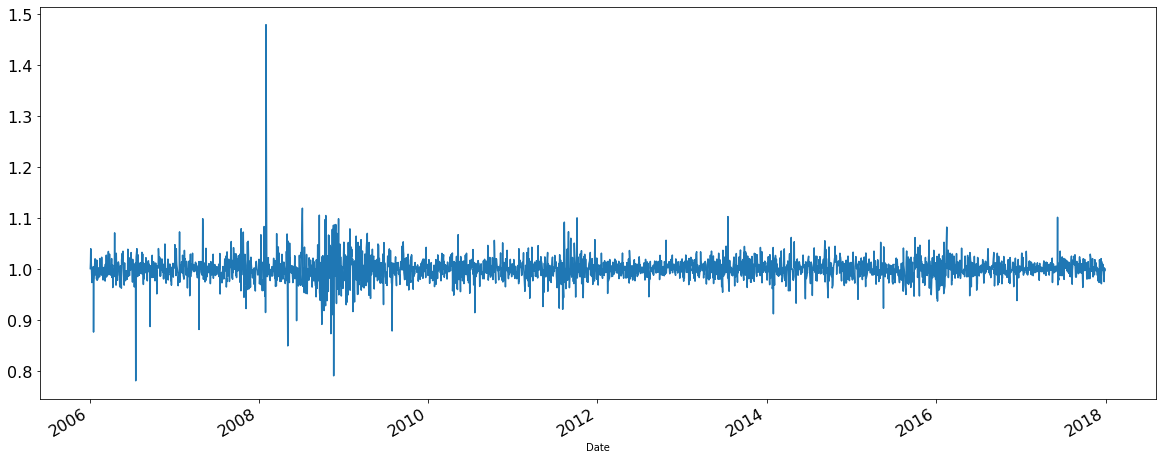You can simply take a specific period and plot to have a clearer look. This is the plot of 2017 only.

`df['2017']['Change'].plot(figsize=(10, 6))`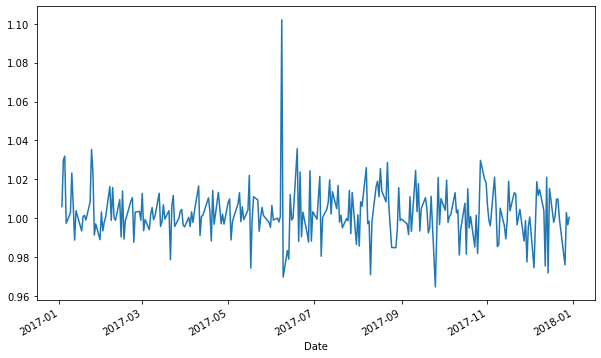Though the shift is useful in many ways. But I find percent change useful on many occasions.

Percent_Change

I will use the monthly data that was calculated in the beginning. This time I chose bar plots. It shows the percent change clearly. There is a percent change function available to get the percent_change data.

`df_month.loc[:, 'pct_change'] = df.Close.pct_change()*100fig, ax = plt.subplots()df_month['pct_change' ].plot(kind='bar', color='coral', ax=ax)ax.xaxis.set_major_locator(mdates.WeekdayLocator())ax.xaxis.set_major_formatter(mdates.DateFormatter('%b %d'))plt.xticks(rotation=45)ax.legend()`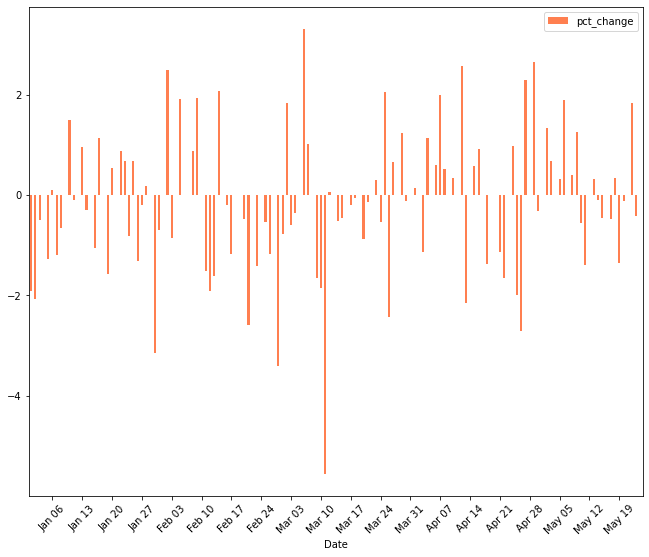I plotted the percent change in closing data here. I used monthly percent change here.

Differencing

Differencing takes the difference in values of a specified distance. By default, it’s one. If you specify 2 like “df.High.diff(2)’, it will take the difference of first and third element of ‘High’ column, second and fourth element, and so on.

It is a popular method to remove the trend in the data. The trend is not good for forecasting or modeling.

`df.High.diff().plot(figsize=(10, 6))`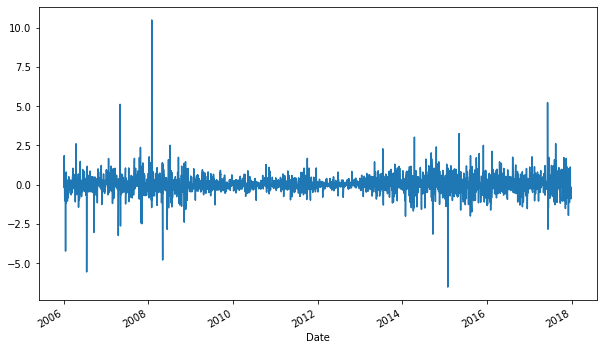Expanding Window

Another way of transformation. It keeps adding the cumulative. For example, if you add an expanding function to the ‘High’ column first element remains the same. The second element becomes cumulative of the first and second element, the third element becomes cumulative of the first, second, and third element, and so on. You can use aggregate functions like mean, median, standard deviation, etc. on it too.

In that way, it will provide you with the changing mean, median, sum, or standard deviation with time. Isn’t it really useful for financial data or business sales or profit data?

`fig, ax = plt.subplots()ax = df.High.plot(label='High')ax = df.High.expanding().mean().plot(label='High expanding mean')ax = df.High.expanding().std().plot(label='High expanding std')ax.legend()`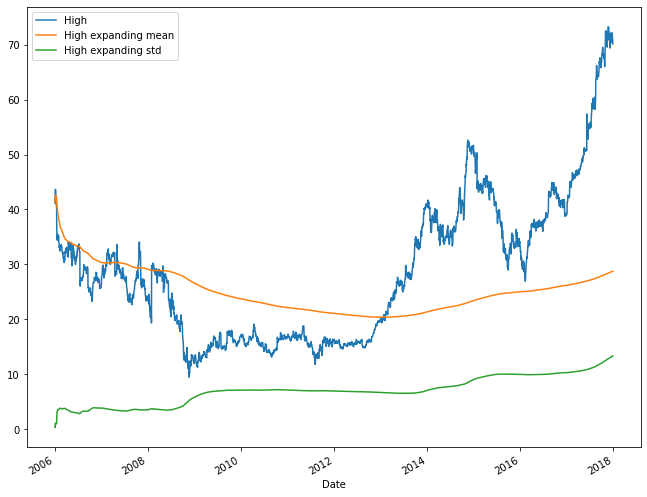Here I added expanding mean and standard deviation. Look at the daily data and the mean. At the end of 2017, daily data shows a huge spike. But it doesn’t show a spike in the average. Probably if you take the 2017 data only, the expanding average will look different. Please feel free to try it.

## Heat Map

A heat map is generally a common type of data visualization that is used everywhere. In time-series data also heat maps can be very useful.

But before diving into the heat map, we need to develop a calendar that will represent each year and month data of our dataset. Let’s see it in an example.

For this demonstration, I will import a calendar package and use the pivot table function to generate the values.

`import calendarall_month_year_df = pd.pivot_table(df, values="Open",                                   index=["month"],                                   columns=["year"],                                   fill_value=0,                                   margins=True)named_index = [[calendar.month_abbr[i] if isinstance(i, int) else i for i in list(all_month_year_df.index)]] # name monthsall_month_year_df = all_month_year_df.set_index(named_index)all_month_year_df`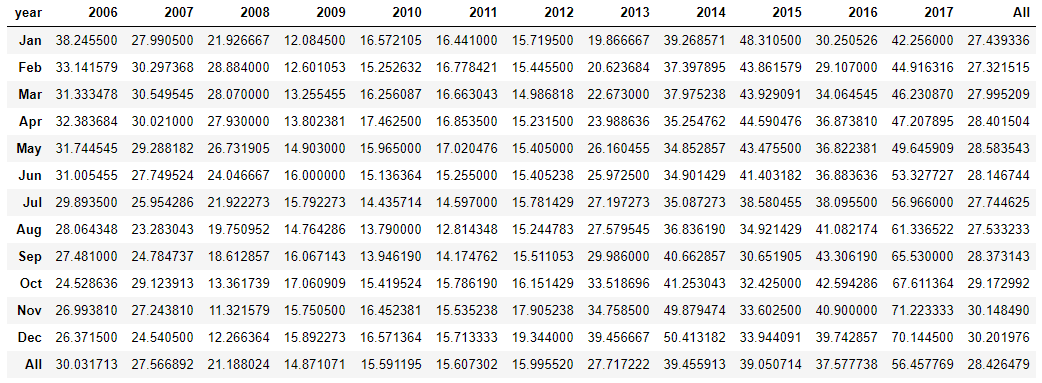The calendar is ready with monthly average ‘Open’ data. Now, generate the heat map with it.

`ax = sns.heatmap(all_month_year_df, cmap='RdYlGn_r', robust=True, fmt='.2f',                  annot=True, linewidths=.5, annot_kws={'size':11},                  cbar_kws={'shrink':.8, 'label':'Open'})                           ax.set_yticklabels(ax.get_yticklabels(), rotation=0, fontsize=10)ax.set_xticklabels(ax.get_xticklabels(), rotation=0, fontsize=10)plt.title('Average Opening', fontdict={'fontsize':18},    pad=14);`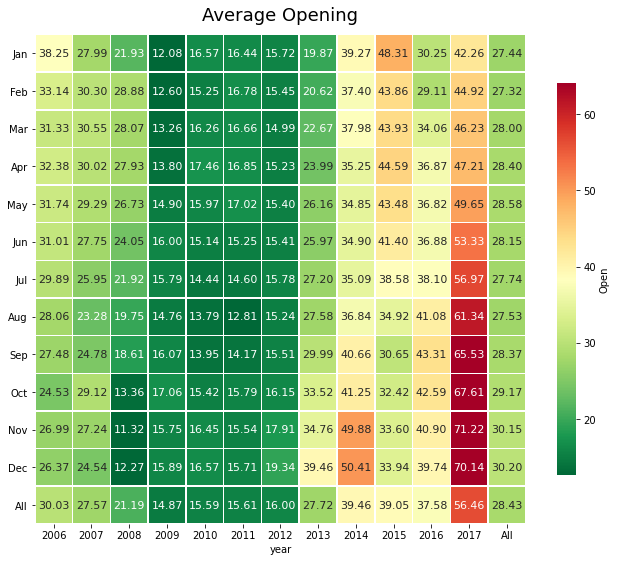The heat map is ready! Darker red means very high opening and dark green mean very low opening.

## Decomposition

Decomposition will show the observations and these three elements in the same plot:

Trend: Consistent upward or downward slope of a time series.

Seasonality: Clear periodic pattern of a time series

Noise: Outliers or missing values

Using the stats model library, it is easy to do it:

`from pylab import rcParamsimport statsmodels.api as smrcParams['figure.figsize'] = 11, 9decomposition = sm.tsa.seasonal_decompose(df_month['Volume'], model='Additive')fig = decomposition.plot()plt.show()`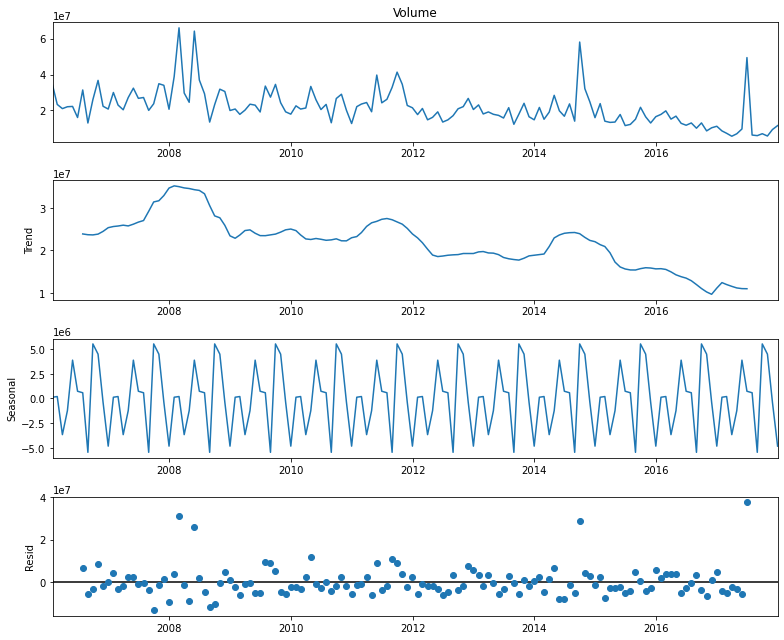Here the trend is the moving average. To give you a high-level idea of residuals in the last row, here is the general formula:

Original observations = Trend + Seasonality + Residuals

Though the documentation for decomposition itself says that it’s a very naive representation but it is still popular.

## Conclusion

If you could run all the code above, Congratulation! You learned enough today to make a great level of time series of data visualization. As I mentioned in the beginning, there are a lot of cool visualization techniques available. I will write more in the future.